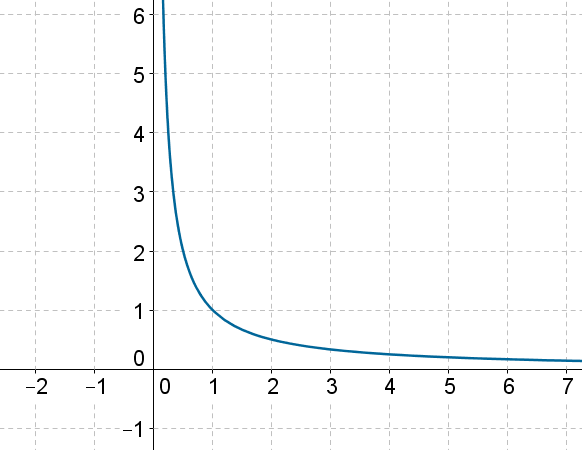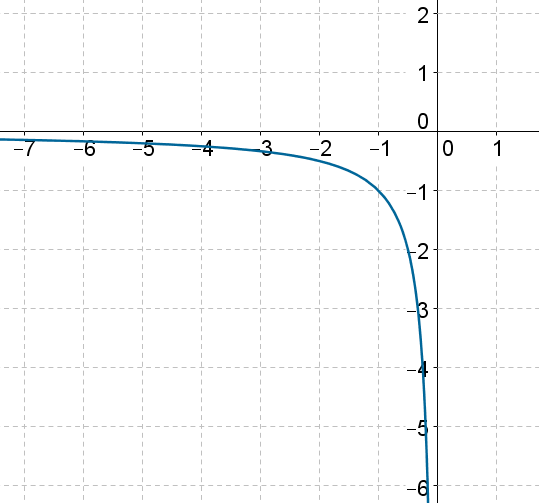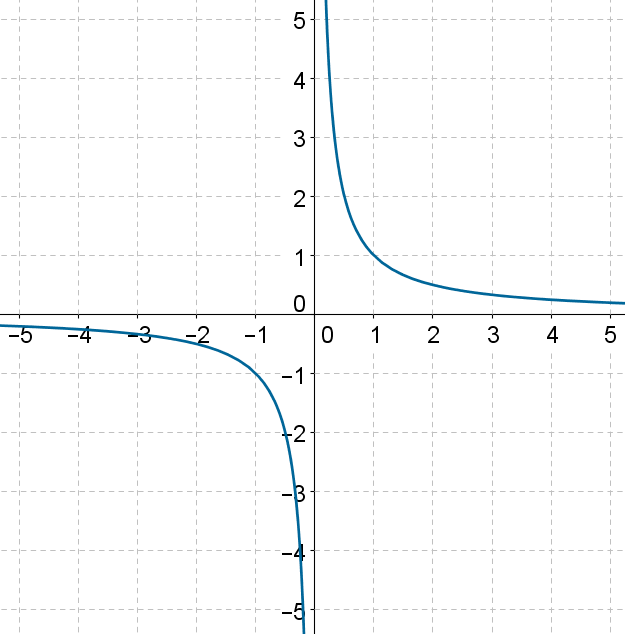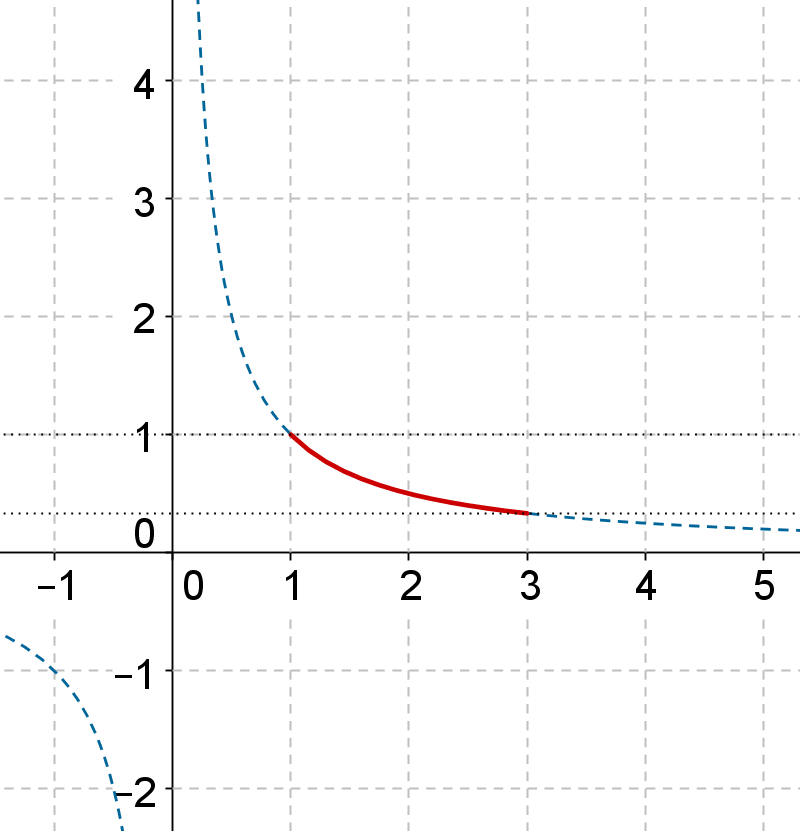# Reciprocal Function

Go back to  'Functions'

The reciprocal function is defined as

$f\left( x \right) = \frac{1}{x}$

The domain of this function is  $$D = \mathbb{R} - \left\{ 0 \right\}$$ . What will be the range of this function?

Let us first analyze the behavior of this function in more detail. The following table shows function output values for some values in $$\left[ {1,\infty } \right)$$:

 x $$f\left( x \right) = \frac{1}{x}$$ 1 1 2 $$\frac{1}{2} = 0.5$$ 10 $$\frac{1}{{10}} = 0.1$$ 100 $$\frac{1}{{100}} = 0.01$$ 1000 $$\frac{1}{{1000}} = 0.001$$ 1 million 0.000001

We can conclude that as increases towards positive infinity, its reciprocal decreases towards zero: as $$x \to \infty$$, $$\frac{1}{x} \to 0$$.

Next, the following table shows functions output values from some values of x in $$\left( {0,1} \right]$$:

 x $$f\left( x \right) = \frac{1}{x}$$ 1 1 0.5 $$\frac{1}{{0.5}} = 2$$ 0.1 $$\frac{1}{{0.1}} = 10$$ 0.01 $$\frac{1}{{0.01}} = 100$$ 0.001 $$\frac{1}{{0.001}} = 1000$$ 0.000001 1 million

Clearly, as $$x \to 0$$, $$\frac{1}{x}\to \infty$$. This is intuitively obvious also: the smaller the magnitude of a number, the larger the magnitude of its reciprocal.

The following figure shows the graph of $$f\left( x \right) = \frac{1}{x}$$  for $$x > 0$$:This is in alignment with our earlier observations.

Now, how will the function behave for negative values of x? Observe the following table:.

 x $$f\left( x \right) = \frac{1}{x}$$ –1 –1 –2 $$- \frac{1}{2} = - 0.5$$ –10 $$- \frac{1}{{10}} = - 0.1$$ –100 $$- \frac{1}{{100}} = - 0.01$$ –1000 $$- \frac{1}{{1000}} = - 0.001$$ –1 million –0.000001

Clearly, the magnitude of decreases as x increases towards negative infinity: as $$x \to - \infty$$, $$\frac{1}{x} \to 0$$.

Let us see that happens in the interval $$\left[ { - 1,0} \right)$$.Observe the following table:

 x $$f\left( x \right) = \frac{1}{x}$$ –1 –1 –0.5 $$- \frac{1}{{0.5}} = - 2$$ –0.1 $$- \frac{1}{{0.1}} = - 10$$ –0.01 $$- \frac{1}{{0.01}} = - 100$$ –0.001 $$- \frac{1}{{0.001}} = - 1000$$ –0.000001 –1 million

Thus, we see that as approaches 0 from the negative side, the value of $$\frac{1}{x}$$ moves towards negative infinity.The following is the plot of $$f\left( x \right)= \frac{1}{x}$$ for $$x < 0$$:The complete graph of $$f\left( x \right) = \frac{1}{x}$$ is now plotted below:Let us summarize our discussion on the reciprocal function $$f\left( x \right) =\frac{1}{x}$$:

1. The domain of this function is  $$\mathbb{R} - \left\{ 0 \right\}$$.

2. As $$x \to \infty$$, $$f \to {0^ + }$$. The plus superscript in $$f \to {0^ + }$$ indicates that approaches 0 from the positive side – in other words, f moves towards 0 but is positive.

3. As $$x \to - \infty$$, $$f \to {0^ - }$$. The minus superscript in $$f \to {0^ - }$$ indicates that approaches 0 from the negative side – in other words, f moves towards 0 but is negative.

4. As $$x \to {0^ + }$$, $$f \to \infty$$. Once again, $$x \to {0^ + }$$ indicates that x moves towards 0 from the positive side.

5. As $$x \to {0^ - }$$, $$f \to - \infty$$.

6. There is a discontinuity in the function’s graph for $$x = 0$$. This is because the function does not have $$x = 0$$ in its domain.

Finally, we see that generates all output values except 0. In other words, there is no value of for which $$\frac{1}{x} = 0$$. For any arbitrary non-zero real value k, if we take $$x = \frac{1}{k}$$, the output k is generated. Thus, every output other than 0 can be generated by f.

This means that the range of $$f\left( x \right) = \frac{1}{x}$$ is  $$\mathbb{R} - \left\{ 0 \right\}$$  .

Example 1: g is a function defined on $$\left[ {1,3}\right]$$ such that $$g\left( x \right) =\frac{1}{x}$$. What is the range of g?

Solution: The following figure shows the graph of $$f\left( x \right) = \frac{1}{x}$$ highlighted for x in the interval $$\left[ {1,3}\right]$$:Clearly, the range of g is $$\left[ {\frac{1}{3},1} \right]$$.

Functions
Functions
grade 10 | Questions Set 2
Functions
Functions
grade 10 | Questions Set 1